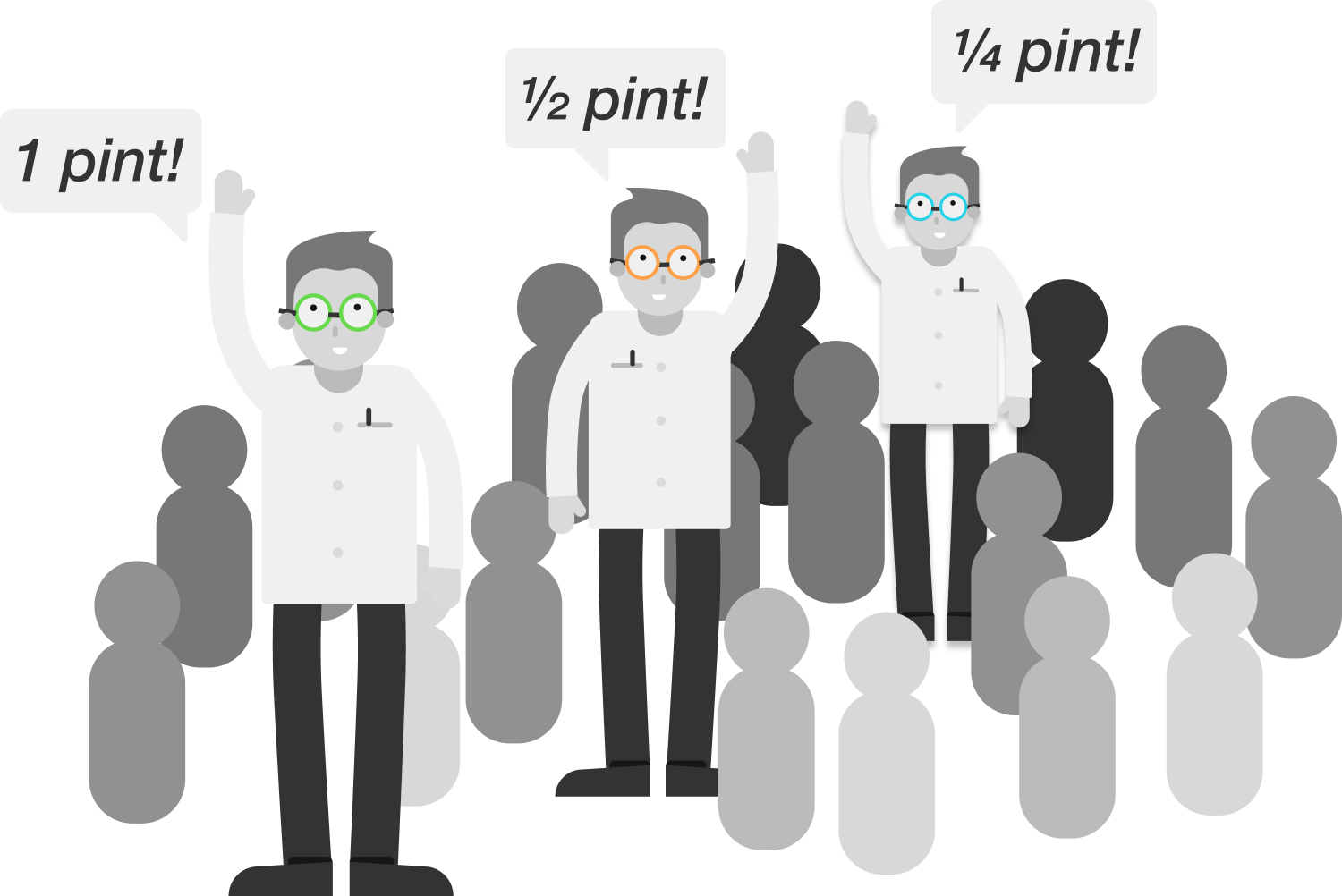# Mathematicians In A Bar

Calculus Level 1An infinite crowd of mathematicians enters a bar. The first one orders a pint, the second one orders a half pint, the third one orders a quarter pint, and so on.

The bartender interrupts, "I get it!" – then pours the order for all of the mathematicians. How many pints does the bartender pour?

×